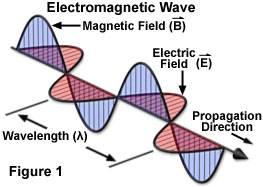# Directional conservation problem with E=cB?

Clavis
As I understand it, the ratio of the electric field strength to the magnetic field strength at any point in a propagating electromagnetic plane wave is equal to the speed of light. How can the electric and magnetic field be perpendicular to each other yet still validate the E = cB equation? A vector (B) multiplied by a scalar (c) should always yield a different size vector (E) in the same direction as the original. I'm thinking the solution may lie with some directional contribution that must be implied with the speed of light corresponding to the wave's propagation direction.

Do you have a reference for your formula? If memory serves me correctly, there is a 90-degrre phase difference between the E and the B field in an EM wave. That would seem to directly disagree with your formula.

Clavis
I agree rumborak that 90 degree phase difference between the E and B field in an EM wave directly disagrees with the E = cB formula. That's precisely the dilemma I'm having. The E = cB equation falls out of the Maxwell's electromagnetism equations when solving for a plane wave though.
http://srjcstaff.santarosa.edu/~lwillia2/42/WaveEquationDerivation.pdf

Your link is correct, but also misleading in the text. By not considering a phase shift between the solution for the E field and the B field (eq 5 and 6), thus effectively setting the phase shift to 0, they consider only what's called a linear wave. For those E/B = c holds. It may be the simplest wave to consider, but there are others (circular, elliptical), for which the E/B = c then no longer holds. The text makes it sound as if it would hold for any kind of wave.
(The circular polarized wave is the one with the 90 degree shift, FYI)

Clavis
Linear electromagnetic prorogations still have their E field and B field 90 degrees from each other as shown in the link. Upon farther scrutiny of said link i posted early, I see that the speed of light represented as c has an arrow above it in the diagram at the top of the paper; this puts the problem to rest because c is being treated as a vector. I didn't realize that was an option until a few minutes ago, but I suppose any speed can be treated as a vector if the direction is known, which it is in this case, the direction being the propagation direction of the wave. I was beginning to suspect that anyway as I initiated this conversation. Thanks for prompting me to think this through.

Yikes, no, that is entirely the wrong conclusion. c is a proportionality factor and has no inherent direction. A wave will travel with a speed of c in a certain direction, but that is different.

Regarding the phases, here another link:

https://sureshemre.wordpress.com/20...ield-oscillations-of-an-electromagnetic-wave/

There is a difference between the *spatial angle* between E and B (which indeed is always 90 degrees) and the phase between them (which can be any value).

Clavis
Hmmm... okay.

I guess I'm confused again then.

If the E field is definitively in a different direction then the B field and c must be constrained as a scalar proportionality constant, then how does E = cB hold true mathematically for a linear E&M wave? Does the phase angle being zero somehow make the unit vectors go away?

anorlunda
Staff EmeritusIn that picture, imagine a photon propagating in the x direction, E field oscillates in the y direction, B field oscillates in the z direction. There is no phase shift.

When many photons travel together, the wave description of their collective behavior is more useful than thinking of them as particles. It depends on the context whether discrete photons or waves are the most useful description.

A wave or beam or ray , may or may not be polarized. 100% Polarized means that the E orientations of all the photons are lined up with each other. 0% means random orientation of the E fields. But for one photon at a time, the picture above applies always.

So to properly state your question, you must specify single photons, or a polarized wave, or an unpolarized wave.

Clavis
I understand that there are many situations where E does not equal cB such as unpolarization, when the phase angle isn't zero, when the wave is cirularly polarized, etc. That's great and all. My question is a mathematical one. For the situations where E DOES equal cB, how does this equation mathematically balance? Is E in the same direction as B in this equation, or is c treated as a vector? Are E and B magnitudes in this equation? Or what?